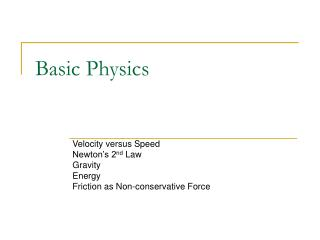# Basic Physics - PowerPoint PPT PresentationDownload PresentationBasic Physics

Basic Physics
Download Presentation## Basic Physics

- - - - - - - - - - - - - - - - - - - - - - - - - - - E N D - - - - - - - - - - - - - - - - - - - - - - - - - - -
##### Presentation Transcript

1. Basic Physics Velocity versus Speed Newton’s 2nd Law Gravity Energy Friction as Non-conservative Force

2. Velocity versus Speed • Pay particular attention when distinguishing between the velocity vector and its scalar magnitude, speed. Which quantity is being used is often implied through context but a distinction should be made. The notational differences are often subtle. • Velocity is the time derivative of the position vector. • Speed is the magnitude of the velocity vector. • Similarly, acceleration is the time derivative of the velocity vector.

3. Newton’s 2nd Law • Newton’s 2nd law of motion states that the acceleration a of an object of constant mass m is proportional to the vector sum of the forces F acting on it. • The 2nd law is also applied to the individual components. • While a non-zero acceleration always implies a change in velocity, it does not always imply a change in speed. How can this be true?

4. Gravitational Forces • Near the surface of the earth, the force of gravity does not vary significantly with height. We assume the force of gravity is constant in the cases we consider in this class. • In a Cartesian system with the j direction pointing up, the gravitational force on mass m is: F = -mgj where g is the magnitude of gravity near the surface of the earth, g=9.81m/s2.

5. Energy (1) • The total mechanical energy of a system is the sum of the kinetic energies (KE) of motion and potential energies (PE) associated with the position of the system in space. • We express kinetic energy in two useful ways: • Translational • Rotational – about the center of mass of the object • There are many sources of potential energy, e.g. • Gravitational • Electrostatic • Magnetic • Spring • Roller coasters deal with both expressions of kinetic energy but traditionally only gravitational potential energy after the lift hill.

6. Energy (2) • Kinetic Energy (KE) • Translational: KE t = ½mv2 • Rotational: KE r = ½Iω2 (m=mass, v=velocity, I=moment of inertia, ω=angular velocity) • Potential Energy (PE) • Gravitational: PE = mgh (g=gravitational acceleration near the earth’s surface, h=height) • Total Mechanical Energy (E) • E = KE t + KE r + PE = ½mv2 + ½Iω2 + mgh

7. Energy (3) • Conservation of Energy (work-energy theorem) • Assuming there is no energy lost to non-conservative forces, the total mechanical energy of the system remains constant. • Friction is the best example of a non-conservative force – more on friction later – but for now, assume no energy lost to friction • Therefore, Ef = Ei where f stands for final, i for initial ½mvf2 + ½Iωf2 + mghf = ½mvi2 + ½Iωi2 + mghi • Example – assume a point mass (so neglect rotation term) • ½mvf2 + mghf = ½mvi2 + mghi • How can you use this relationship if you know that the initial velocity is zero and the final height is zero?

8. Friction • Friction is a non-conservative force. By doing work on the system, friction changes the total mechanical energy. • The magnitude of the force of friction (f) is found by: • f = μN μ = coefficient of friction, N = normal force • The direction of f is always opposite to the direction of motion • There are three types of friction • Static (μs) - no motion or sliding • Kinetic (μk) - sliding or slipping motion • Rolling (μr) - type of static friction, assuming the ball isn’t slipping as it rolls; the inertia of the ball keeps the ball rolling so the frictional force is due only to deformations or sticking of the two surfaces and is significantly less than the regular coefficient of static friction • There is a difference between neglecting friction and a negligible frictional force.